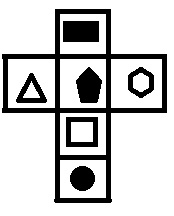# Symbol Sequence Online Quiz

Following quiz provides Multiple Choice Questions (MCQs) related to Symbol Sequence. You will have to read all the given answers and click over the correct answer. If you are not sure about the answer then you can check the answer using Show Answer button. You can use Next Quiz button to check new set of questions in the quiz.Q 1 -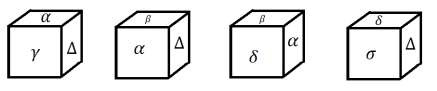Which symbol will face opposite to `α`?

Options :

A - Δ

B - σ

C - δ

D - γ

### Explanation

From the figure, it is clear that δ, γ, Δ, β can't be opposite to the symbol `α`.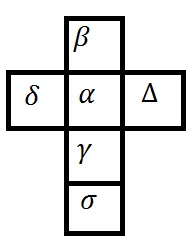Four positions of a dice has been shown in the figures. Answer the questions according to the information provided by figures.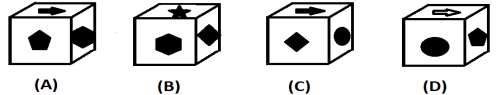Q 2 - Which symbol is on the opposite of face with pentagon?

Options :

A - Rhombus

B - Star

C - Circle

D - Arrow

### Explanation

From the figures (B) and (C), it is clear that circle, star arrow, and hexagon will be on the adjacent face of rhombus which indicates that pentagon must be the opposite of rhombus.

Q 3 -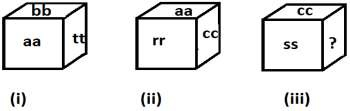Which of the following can be placed in place of question mark ?

Options :

A - ss

B - rr

C - aa

D - tt

### Explanation

Drawing the unfold figure of dice according to the question figure `tt’ can get the place of `?’.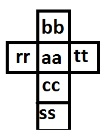Three views of a dice have been shown referring to which give the answer of various questions given below.

Q 4 -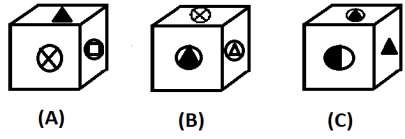The pair of figures that lies adjacent to the face with figure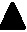is,

Options :

A -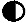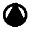B -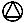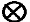C -D - None of these

### Explanation

From the given figure, it can be easily analyzed that ,,,, and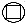will be on the adjacent face oftherefore from given option only option (a) representing the pair of adjacent face.

Q 5 -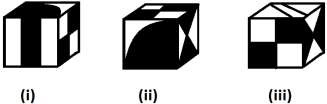Which figure is opposite to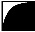if one face has no figure?

Options :

A -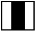B -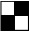C -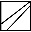D -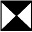### Explanation

From the unfold figure of dice drawn according to the question figure, it is clear thatis opposite to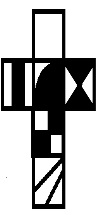Q 6 -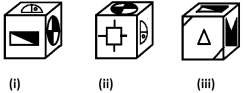Which figure lies opposite to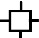?

Options :

A -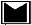B -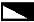C -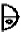D - None of these

### Explanation

From the figures (i) and (iii), it is clear that,,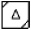and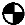will lie on the adjacent face ofwhich clearifies thatmust lie opposite to.

Q 7 -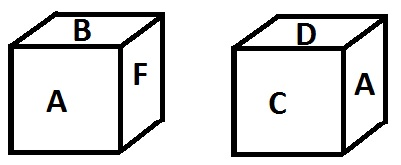Which letter will be opposite of `F’?

Options :

A - A

B - C

C - D

D - B

### Explanation

Various letters on opposite faces can be obtained by equating the letter which occurs more times in the question figure as A = C, D, B, F. Hence on opposite of `A’, the letter will be `E’ therefore following figure can be drawn easily.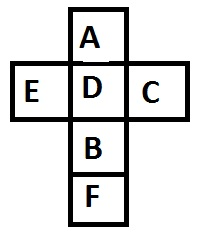∴ Pair of opposite faces are A-E, B-C, and D-F.

Q 8 -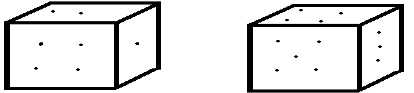In the given figure, two positions of a dice has been shown. Which face will be opposite to the face of 6?

Options :

A - 2

B - 3

C - 4

D - 5

### Explanation

Using the question figure, the unfold figure of dice can be drawn as,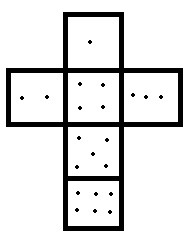### Explanation

By drawing the unfolded figures of the question figure, the answer figure can be found.

On the six faces of dice, various figures have been made which are triangle, rectangle, square, circle, pentagon and hexagon respectively accordingly to the following statements.

Statements :

(i) Triangle is not on the opposite face of pentagon.

(ii) Rectangle and square are not adjacent to each other.

(iii) Pentagon is adjacent to hexagon, square, and rectangle.

(iv) Circle adjacent to square and hexagon.

Q 10 - Beside hexagon, square, and rectangle, which figure is also adjacent to pentagon?

Options :

A - Triangle

B - Circle

C - Square

D - Hexagon